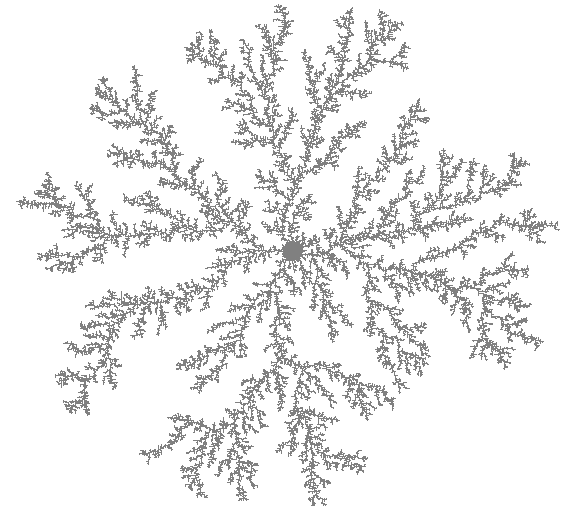My research interest is in theoretical and applied probability, focusing on models motivated from biological and computer sciences and statistical physics. I work on two different areas: self organizing processes (DLA and border aggregation models) and reinforced random processes (like urn models, reinforced random walks).

### Border aggregation model and Diffusion limited aggregationBorder aggregation on circle in $\mathbb{Z}^2$Diffusion limited aggregation in $\mathbb{Z}^2$

Levine and Peres (2007) introduced this model as the internal erosion model, which we later renamed as the Border Aggregation model (see the images above). How long does it take for the arms to reach the origin? How many arms are there? These are some of the most interesting open questions. A major open question is whether the border aggregation can be coupled with diffusion limited aggregation (DLA). There are many interesting questions yet to be settled in this field.

### Urn modelsRandom trees

Urn models form one of the major building blocks in studying random reinforced processes. One of the major applications of these are in clinical trials. These are also applicable in computer science, because of their close connection to random trees. Depending on the type of reinforcement, linear or super-or sub-linear, the finite colour model exihibits phase transitions. In the infinite colour case, are there similar phase transitions? The linear model is closely related to random recursive trees, what can we say about the non-linear case? What is the class of random trees that we can couple with urn models? With many open questions, this is an active area of research.